# Introduction to medical terminology

Nov. 2, 2011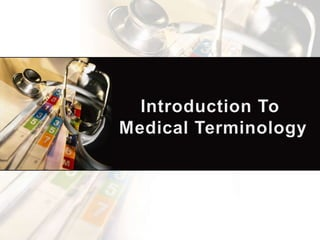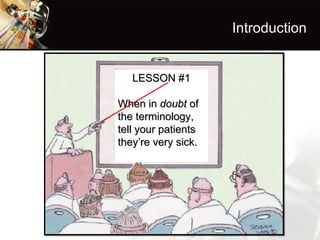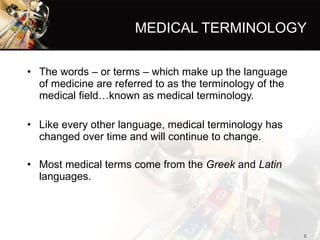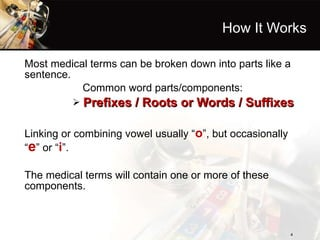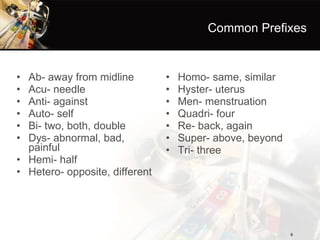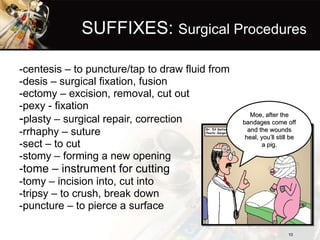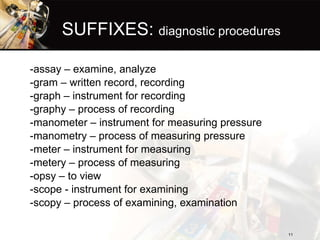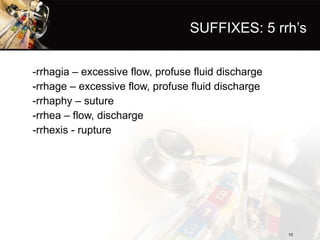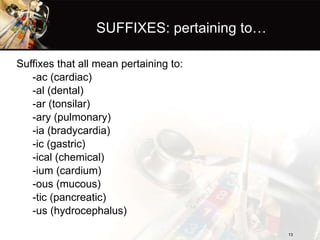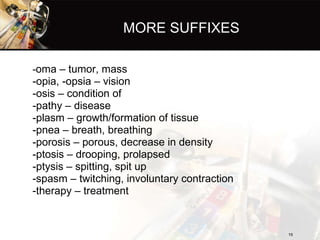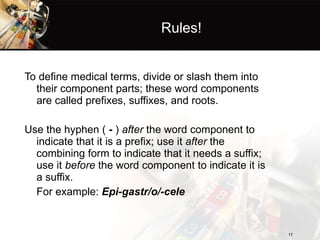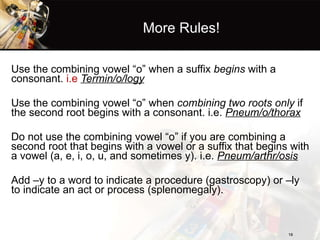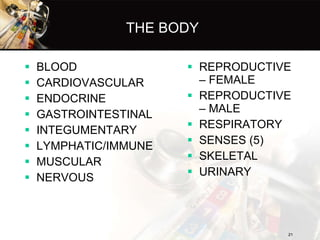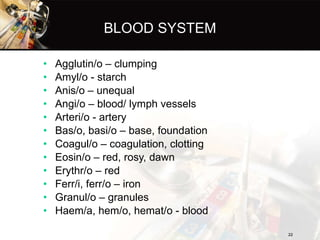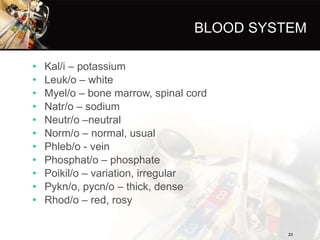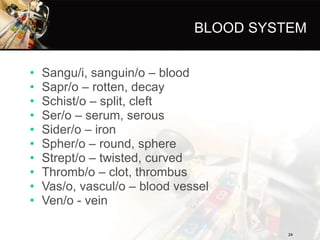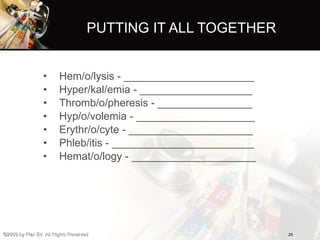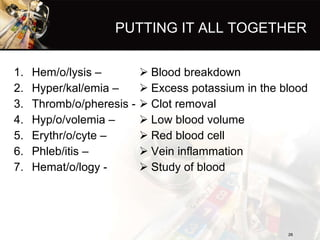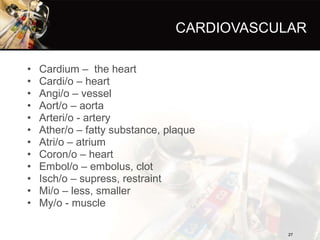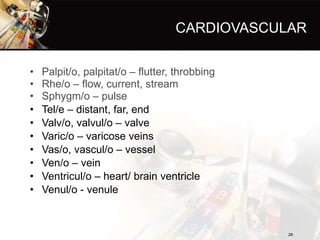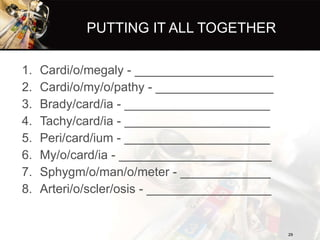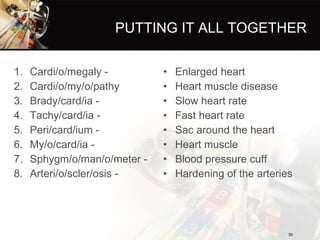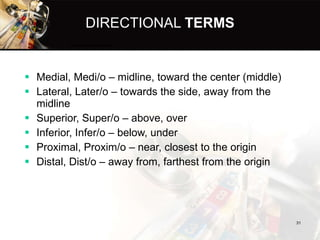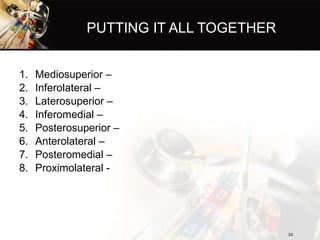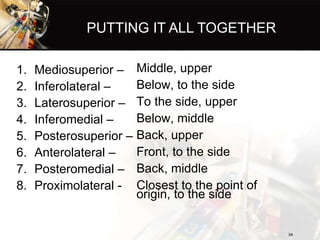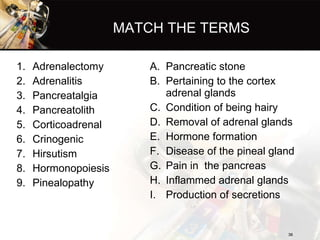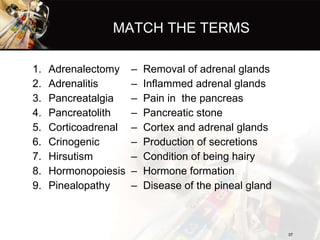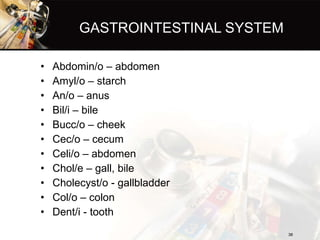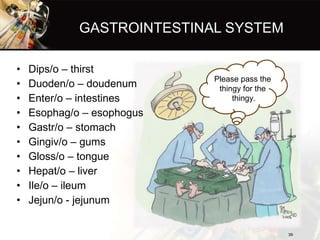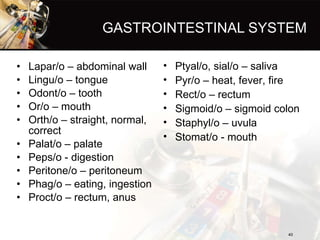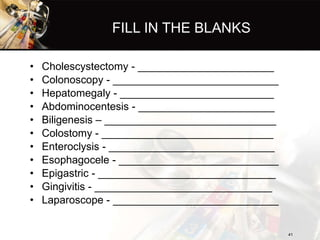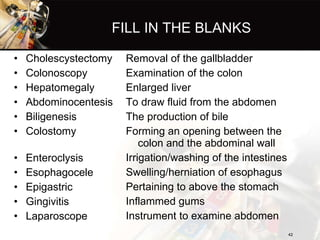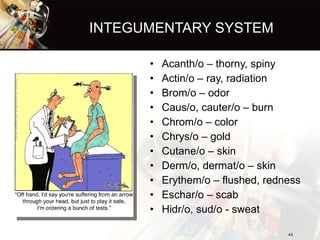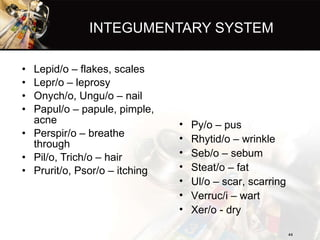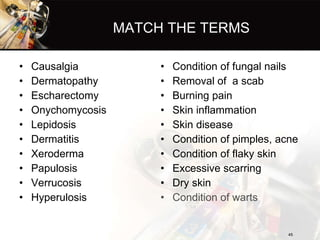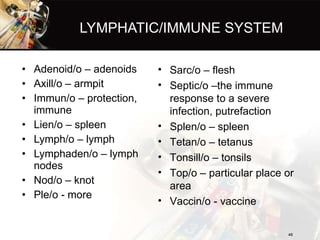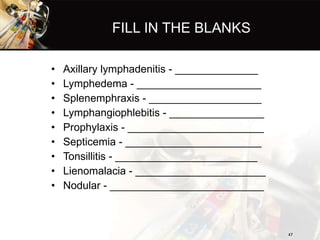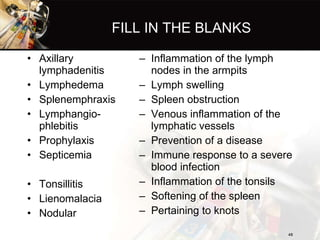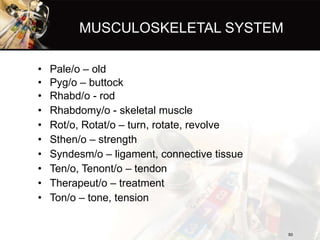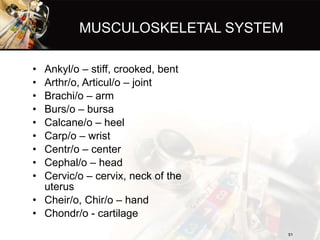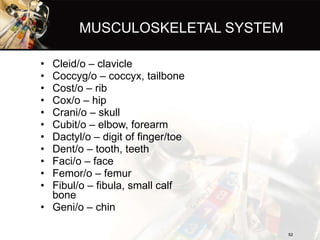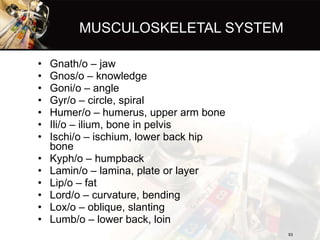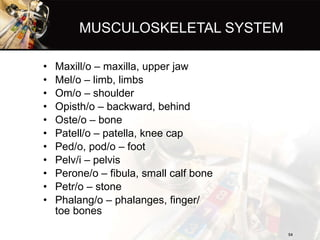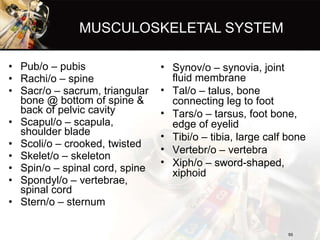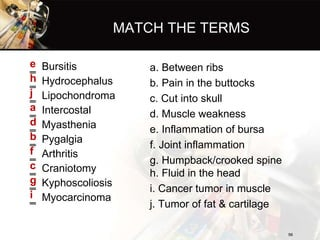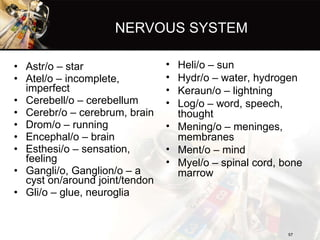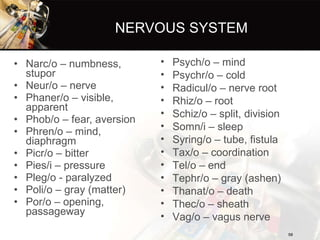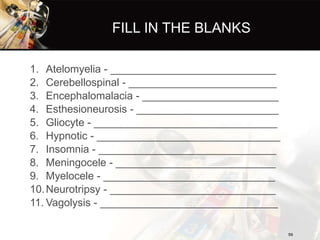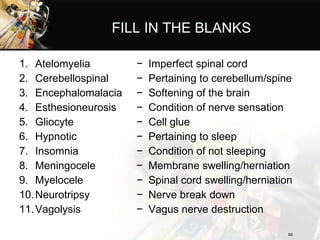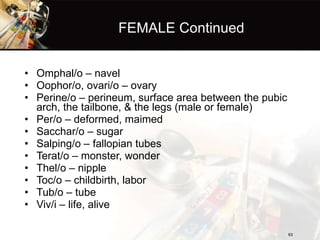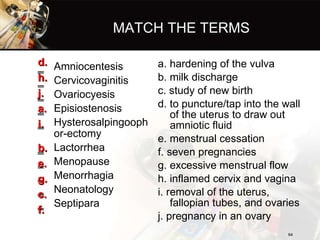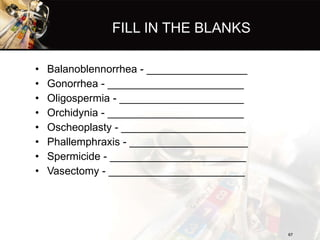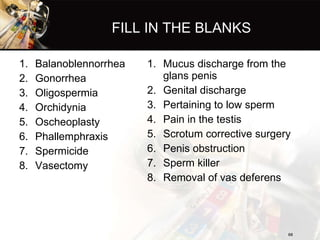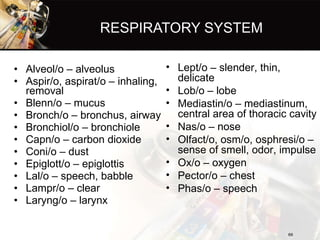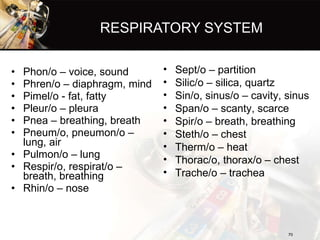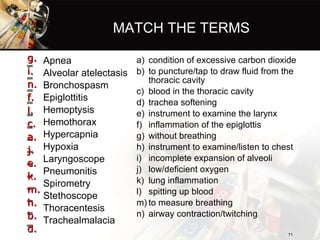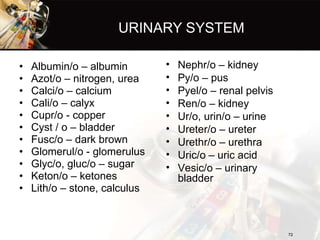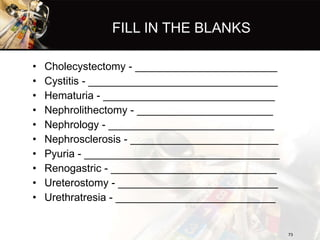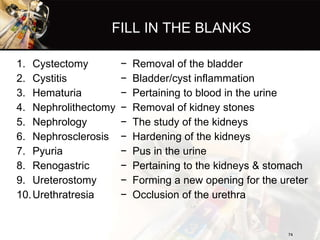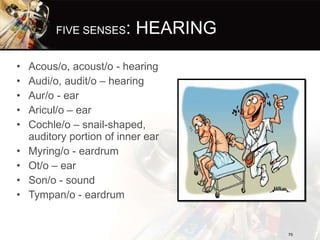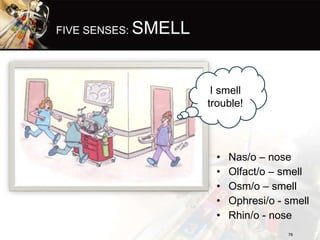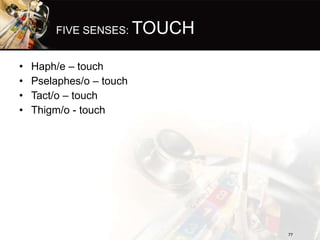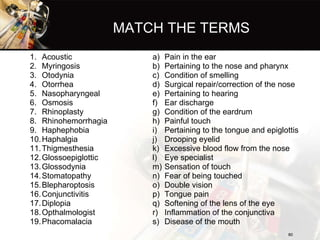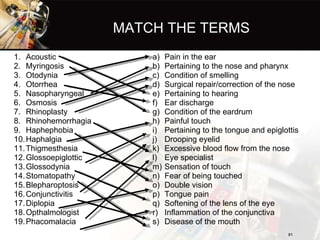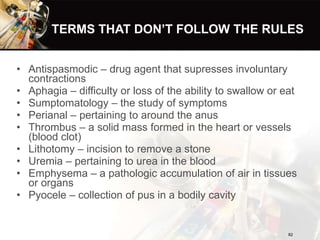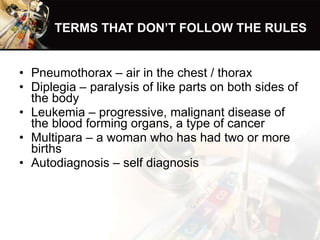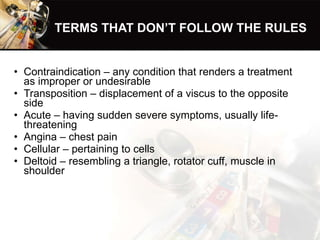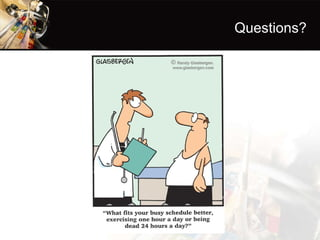1 of 85

### Introduction to medical terminology

• 1.
• 2. Introduction LESSON #1 When in doubt of the terminology, tell your patients they’re very sick.
• 3.
• 4.
• 5.
• 6.
• 7.
• 8.
• 9.
• 10.
• 11.
• 12.
• 13.
• 14.
• 15.
• 16.
• 17.
• 18.
• 19.
• 20.
• 21.
• 22.
• 23.
• 24.
• 25.
• 26.
• 27.
• 28.
• 29.
• 30.
• 31.
• 32.
• 33.
• 34.
• 35.
• 36.
• 37.
• 38.
• 39.
• 40.
• 41.
• 42.
• 43.
• 44.
• 45.
• 46.
• 47.
• 48.
• 49.
• 50.
• 51.
• 52.
• 53.
• 54.
• 55.
• 56.
• 57.
• 58.
• 59.
• 60.
• 61.
• 62.
• 63.
• 64.
• 65.
• 66.
• 67.
• 68.
• 69.
• 70.
• 71.
• 72.
• 73.
• 74.
• 75.
• 76.
• 77.
• 78.
• 79.
• 80.
• 81.
• 82.
• 83.
• 84.
• 85. Questions?

### Editor's Notes

1. Can anyone think of any other everyday terms?
2. STUDENT PAGE
3. POWER POINT ONLY
4. Student page
5. Power point only
6. Student page
7. Power point only
8. Student page
9. Power point only
10. Student page
11. Power point only
12. Student page
13. Student page
14. Power point only
15. Power point only
16. Student page
17. Power point only
18. Power point only
19. Student page
20. Power point only
21. Student page
22. Student only
23. Power point only
24. Student page
25. Power point only